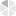## Wednesday, October 16, 2019

Well, today we are going to learn some simple yet very necessary operations of MS Excel. We will teach you a very beginner level thing- “How to perform Basic Arithmetic Calculation in MS-Excel”, so if already know how to do that then this tutorial isn’t for you, just leave this tutorial and find some other tutorial lesson for your need.

Without furthermore delay, let’s proceed to the task;

First you need to open a Blank Workbook from your MS Excel software and it doesn’t really matter which version you are using right now. These arithmetic operations are the fundamental thing of Excel so it will be same for any version of it. These operations are simply based on the formula of addition, subtraction, multiplication and division. What is a formula? This question may arise in your mind. Well, for your kind information, formula is nothing but a simple expression which can calculate values in a cell or in a range of cells.
E.g. 1+2+3=6, right?
In an Excel workbook, every value or anything contains a cell and cell is nothing but the combination of a row and a column. For your clarification,

Then, just enter any value in cells and hopefully, you know how to write values in Excel. If you are not sure then check the below image first,

Then, let’s perform Addition operation first so that you can get an idea how those operations actually work. As you can see from the screenshot, there are three values in the second row so A2=1, B2=2 and C2=3. And, for Addition, we can just write a formula for those cells (A2+B2+C2) and we will get the final result. So, where we are going to write that formula for the Addition. Well, we will perform the Addition operation and write the formula in Addition entitled header in the same row of the values.

To do that, we will write the formula same as the screenshot given here, start with a “ = “ then write the cell numbers or drag your mouse curser to the A2 cell then put a “ + “ symbol then click the B2 cell and again put a “ + “ symbol and click C2 cell.

See the below screenshots,

and you will get the final result of those values in that table. E.g. see the screenshot for the final result of that formula,Hope, it will make some sense to you and you’ve got some idea how actually formula works and how to write them in Excel.
For subtraction, multiplication and division working principle will be same as addition. Just use the right sign for the right operation to occur.
Check formula of those values and cells, for subtraction, multiplication and division in the below gif screen,

Now, for the values of next row, we can simply calculate those basic arithmetic operation. Those values are in cell A3=4, B3=5 and C3=6.
To do addition, subtraction, multiplication and division just drag your mouse to the value of Addition column (here is 6) then press SHIFT key of your keyboard and click your mouse to the last point of values, in this case, Division column and the value of it = 0.1667

You will be able to see such screen,

And, then drag your mouse to the point marked in the image here,

You will see a black ‘+’ symbol, just the drag your mouse to the next row and you will get all the result of the below row entirely.

Hope, this is a very beginner level tutorial which may help you to understand how Excel formula and basic arithmetic calculation works. I suggest you to follow those steps and carefully check the screenshots which are given here.

For your clear understanding about formula and arithmetic operation, we will create a student report card in next tutorial and hopefully, it will get rid of all your confusion.

That’s all for today. Stay with tutorialabc.com for more Excel lessons and always keep practicing. Thanks for your support.